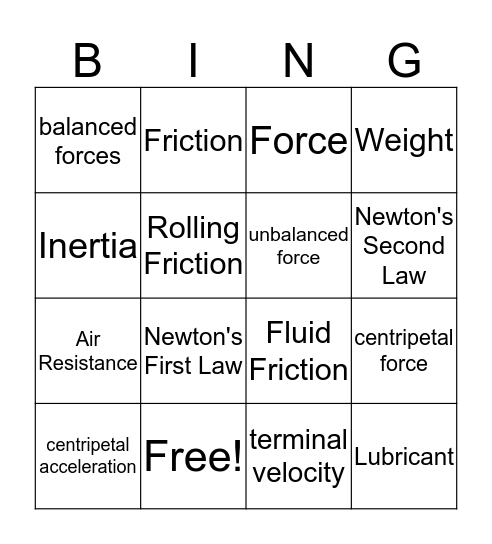# Chapter 12 BingoThis bingo card has 22 words: Free!, Law of conservation of momentum, Friction, Sliding friction, Rolling Friction, unbalanced force, balanced forces, Force, Fluid Friction, Lubricant, Air Resistance, Weight, Gravity, Static Friction, terminal velocity, Net force, centripetal force, centripetal acceleration, Newton's First Law, Inertia, Newton's Second Law and Newton's Third Law.

## Play Online